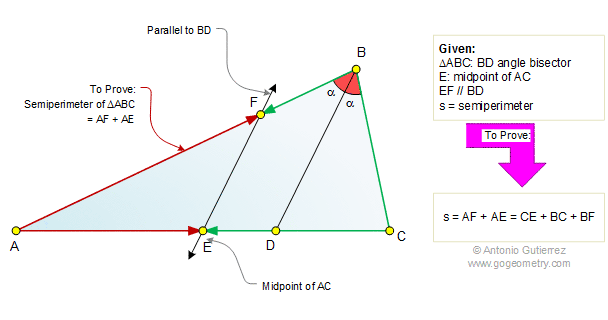# Geometry Problem 531: Triangle, Angle Bisector, Midpoint, Parallel, Perimeter, Semiperimeter

 The figure shows a triangle ABC of semiperimeter "s" with the angle bisector BD. If E is the midpoint of AC and EF is parallel to BD, prove that s = AF + AE = CE + BC + BF.Home | Search | Geometry | Problems | All Problems | Visual Index | 531-540 | Semiperimeter | Email | Post a comment or solution | by Antonio Gutierrez